Homework Help Question & Answers

# 2. Determine the vertical displacement at joint B and horizontal displacement at joint D using Castigliano's...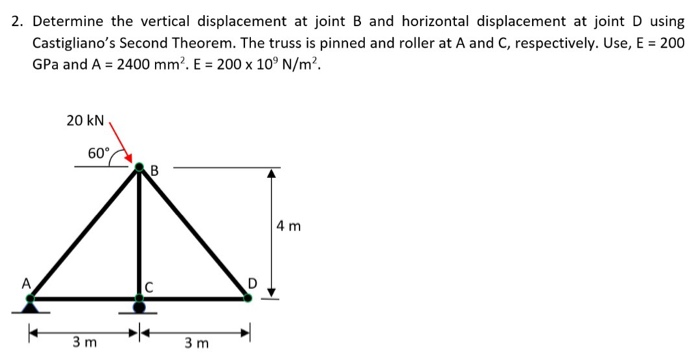2. Determine the vertical displacement at joint B and horizontal displacement at joint D using Castigliano's Second Theorem. The truss is pinned and roller at A and C, respectively. Use, E = 200 GPa and A = 2400 mm. E = 200 x 10°N/m². 20 KN 60 CAB 4 m 3m 3m

#### Homework Answers

Answer #1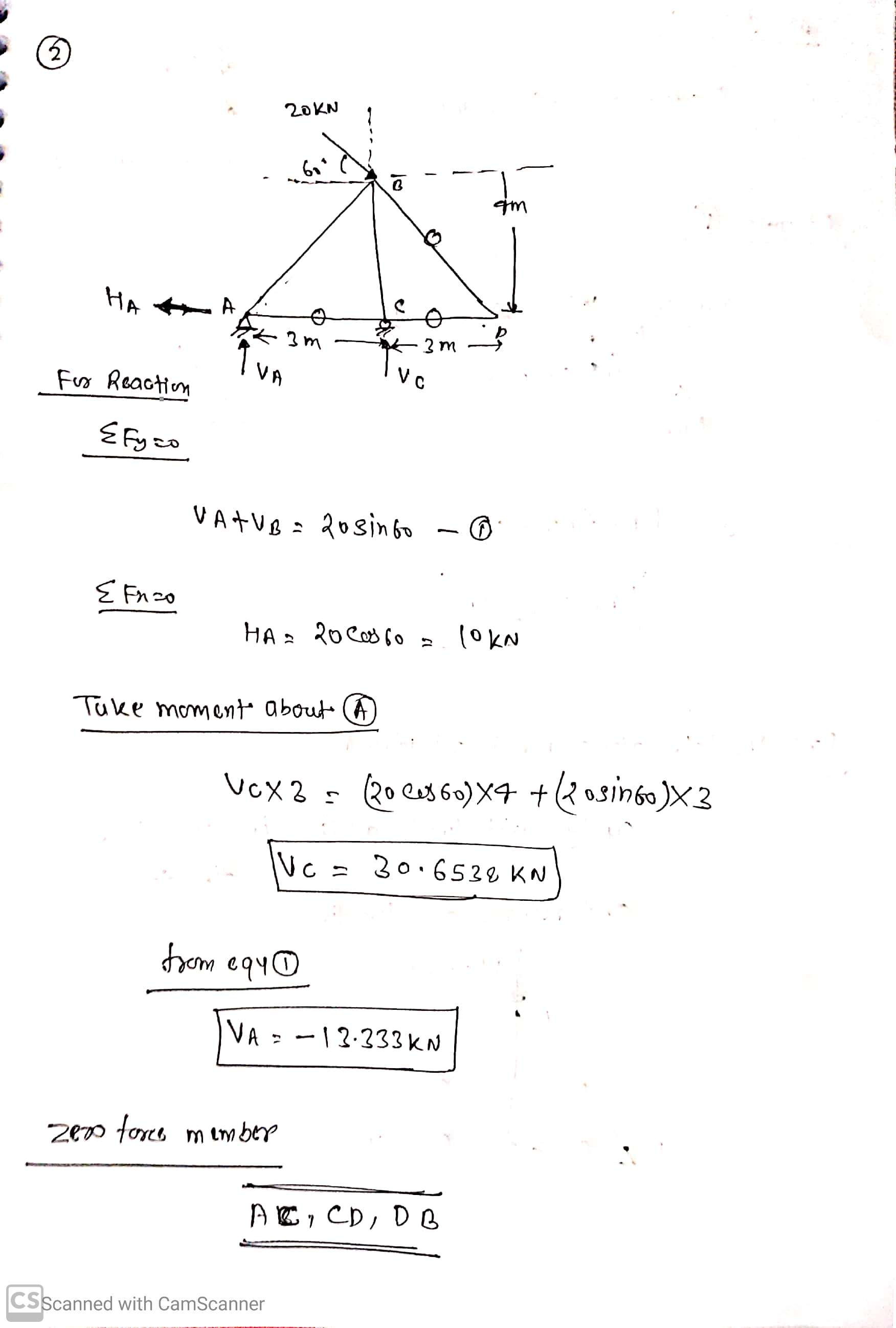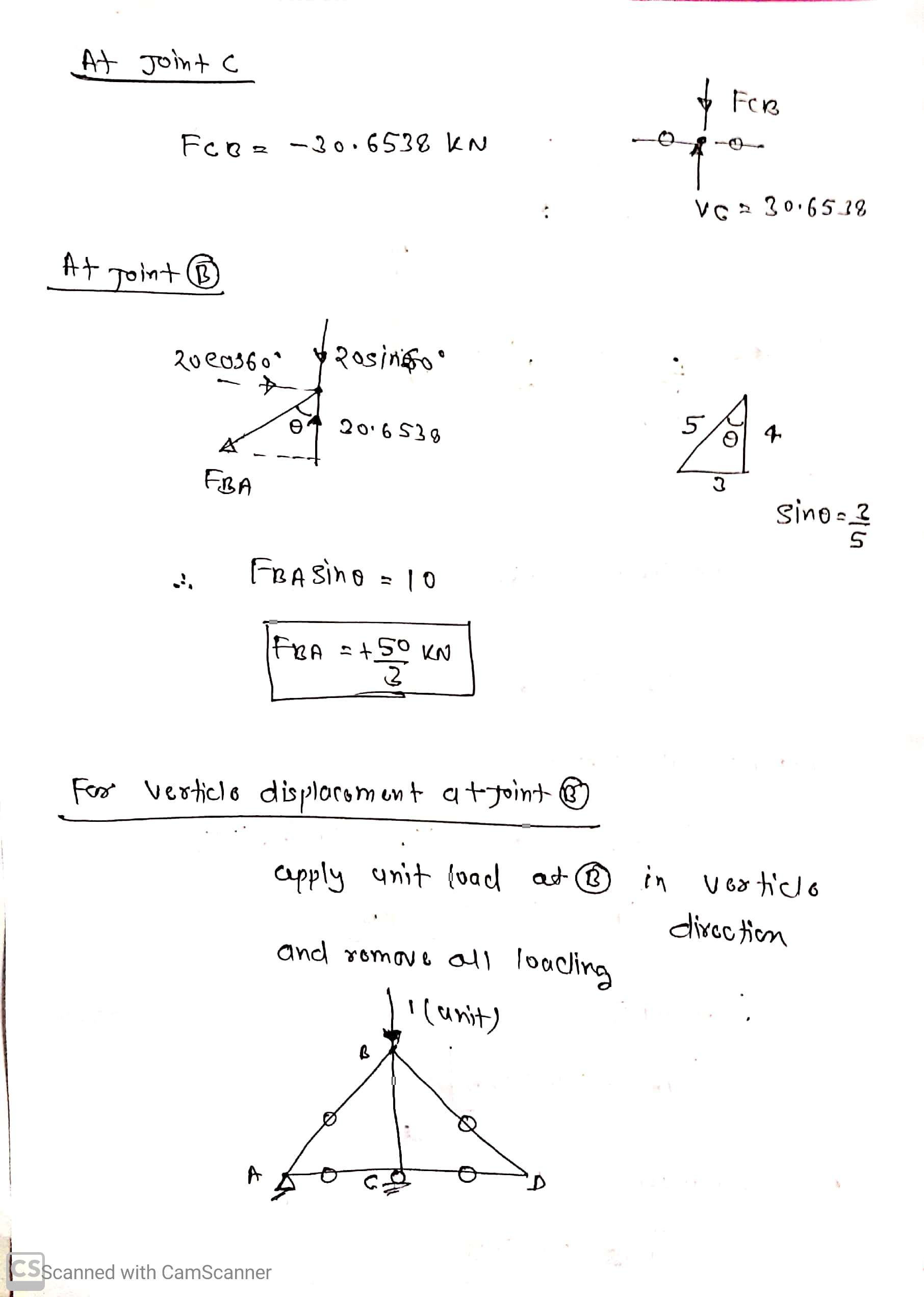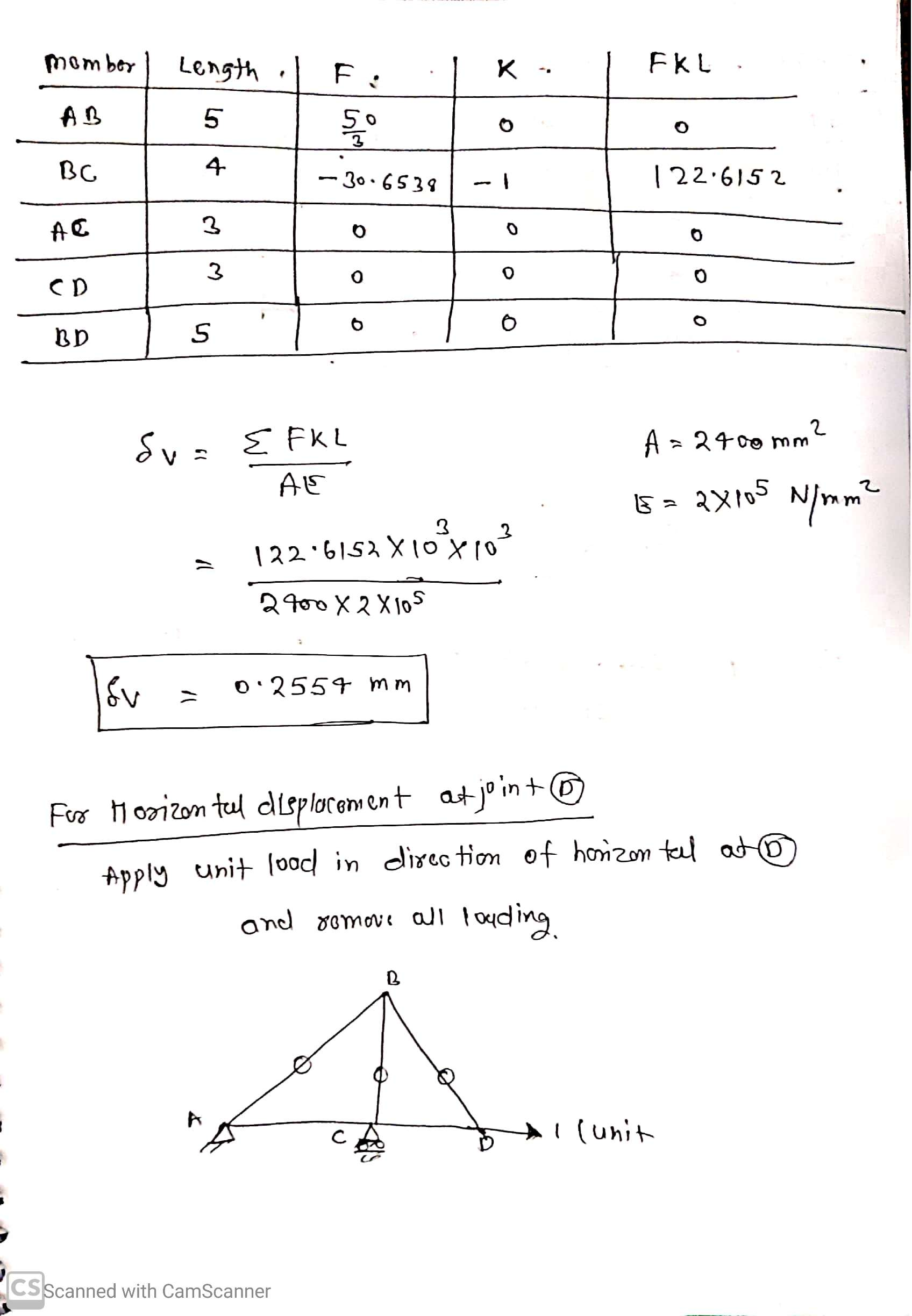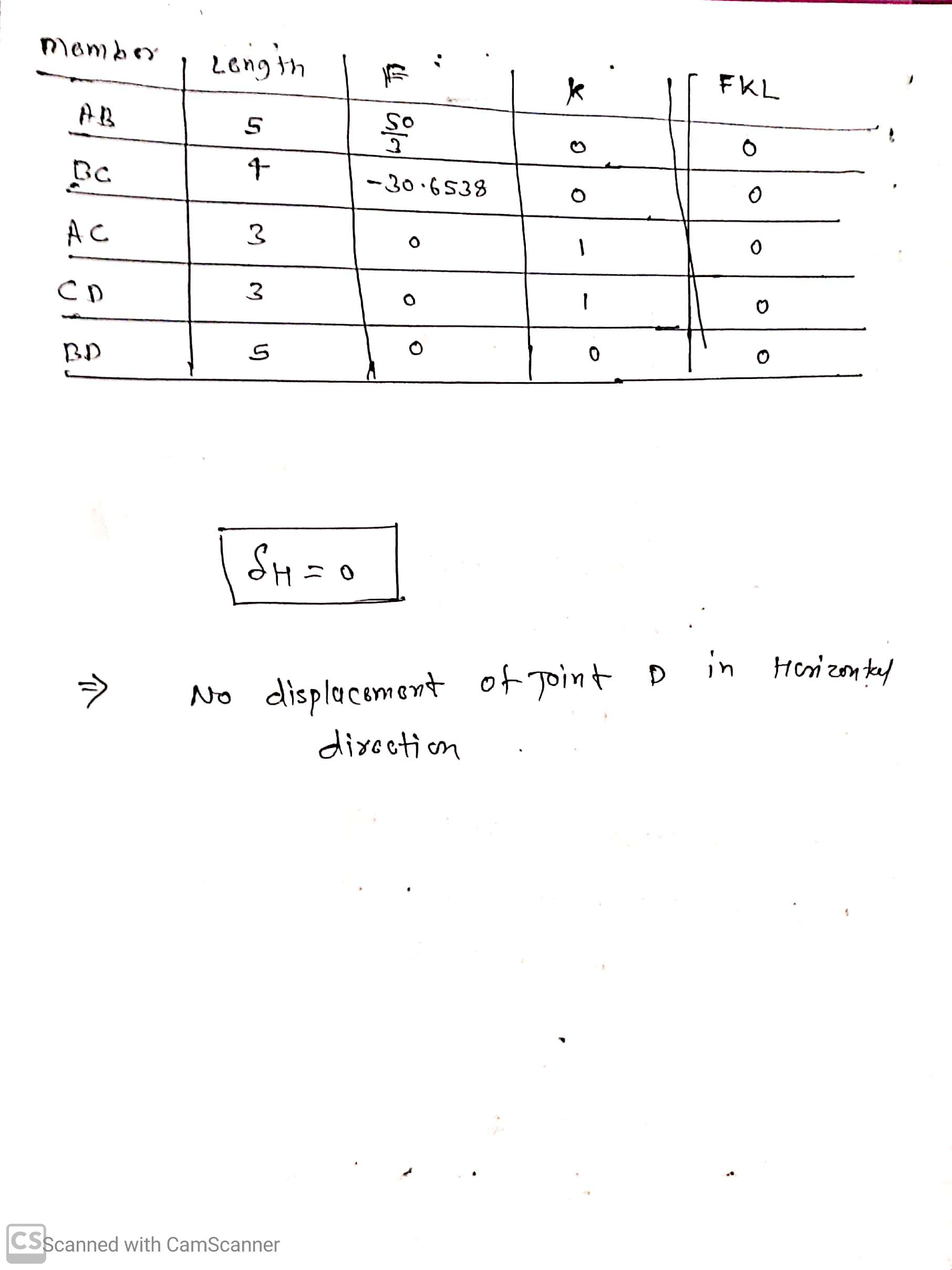Know the answer?
Your Answer:

#### Post as a guest

Your Name:

What's your source?

#### Earn Coin

Coins can be redeemed for fabulous gifts.

Not the answer you're looking for? Ask your own homework help question. Our experts will answer your question WITHIN MINUTES for Free.
Similar Homework Help Questions
• ### Q3: Determine the vertical and horizontal displacement of joint A for the truss shown in Fig....Q3: Determine the vertical and horizontal displacement of joint A for the truss shown in Fig. (3). each bar is made of steel and has the cross-sectional area of 400mm Take E = 200 GPa Use the method of virtual work. E D 2 m |в -1.5 m -1.5 m 20 KN 40 KN Fig. (3)

• ### Q3: Determine the vertical and horizontal displacement of joint A for the truss shown in Fig....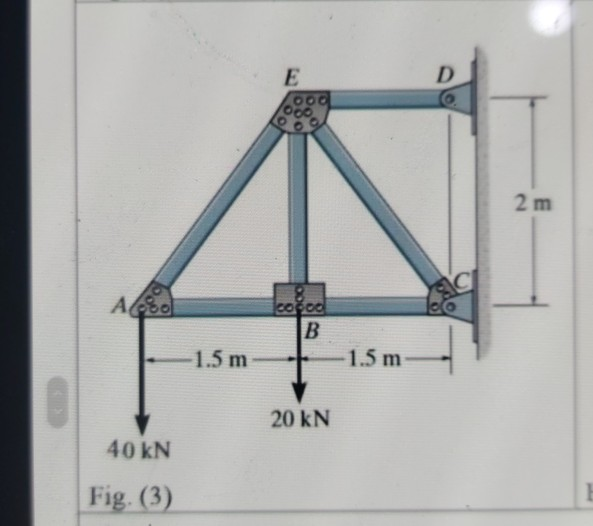Q3: Determine the vertical and horizontal displacement of joint A for the truss shown in Fig. (3), each bar is made of steel and has the cross-sectional area of 400mm?. Take E = 200 GPa. Use the method of virtual work. E D 00. 2 m СІ Ao cocoa B 1.5 m 1.5 m 20 KN 40 kN Fig. (3)

• ### 4. Determine the vertical displacement of point C using Castigliano's theorem. Include axial displacements. Assume E,...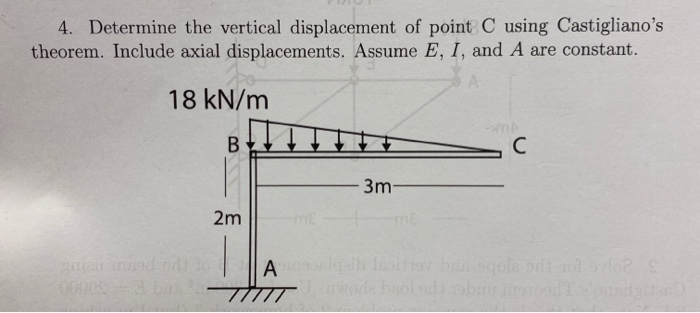4. Determine the vertical displacement of point C using Castigliano's theorem. Include axial displacements. Assume E, I, and A are constant. 18 kN/m 3m- 2m Alle

• ### Solve It fast Please Q3: Determine the vertical and horizontal displacement of joint A for the...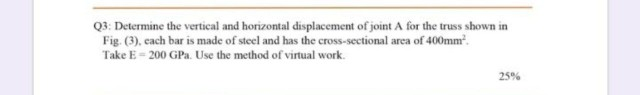Solve It fast Please Q3: Determine the vertical and horizontal displacement of joint A for the truss shown in Fig. (3). cach bar is made of steel and has the cross-sectional area of 400mmº. Take E = 200 GP. Use the method of virtual work. 25% E OO D 2 m AAS ered B -1.5 m -1.5 m 20 KN 40 KN Fig. (3)

• ### 2. (20 points) Using the unit load method (virtual work), find the horizontal displacement of node...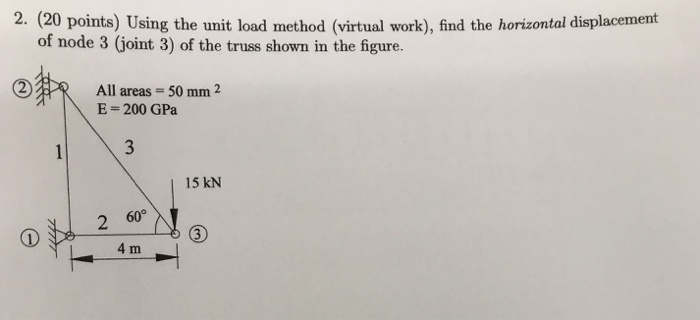2. (20 points) Using the unit load method (virtual work), find the horizontal displacement of node 3 (joint 3) of the truss shown in the figure. ? R All areas = 50 mm 2 E = 200 GPa 15 kN 2 60 L4 m

• ### i need the answer quickly fast as you can Q3: Determine the vertical and horizontal displacement...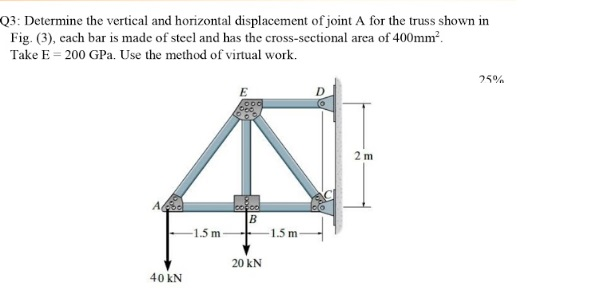i need the answer quickly fast as you can Q3: Determine the vertical and horizontal displacement of joint A for the truss shown in Fig. (3), cach bar is made of steel and has the cross-sectional area of 400mm? Take E = 200 GPa. Use the method of virtual work. 25% E 2 m А. cool B 1.5 m -1.5 m 20 KN 40 KN

• ### Question 1 (25 points) Determine the horizontal displacement of point C. Assume the members are pin...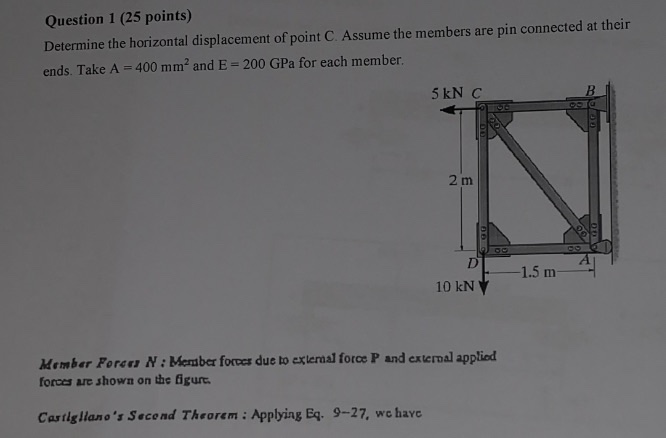Question 1 (25 points) Determine the horizontal displacement of point C. Assume the members are pin connected at their ends. Take A = 400 mm? and E = 200 GPa for each member. 5 kN C 2 m 1.5 m 10 kN Member Foron N: Member forces due to external force P and external applied forces are shown on the figurt. Castigliano's Second Theorem : Applying Eq. 9-27, we have

• ### Consider vertical upward and horizontal to right-hand side to be positive orientations. The below truss is...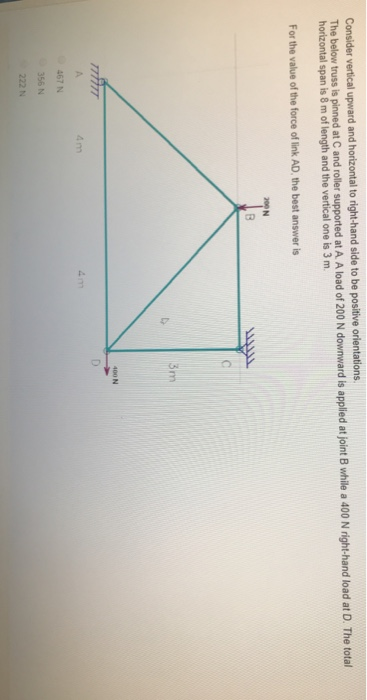Consider vertical upward and horizontal to right-hand side to be positive orientations. The below truss is pinned at C and roller supported at A. A load of 200 N downward is applied at joint B while a 400 N right-hand load at D. The total horizontal span is 8 m of length and the vertical one is 3 m. For the value of the force of link AD, the best answ er is 200 N 3m 400 N D A...

• ### Castigliano’s Theorem Determine the horizontal and vertical displacement at point E (in millimeters). E = 200...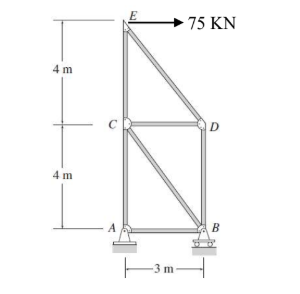Castigliano’s Theorem Determine the horizontal and vertical displacement at point E (in millimeters). E = 200 GPa. All the bars have an area of 5000 mm2 75 KN 4 m 4 m ITm

• ### Use Castigliano's theorem and determine the vertical deflection at C. Include the effects of...

The bent road has an E=200 GPa, G=75 GPa, and a radius of 30 mm. Use Castigliano's theorem and determine the vertical deflection at C. Include the effects of bending,shear, and torsional strain energy.

Free Homework App

Scan Your Homework
to Get Instant Free Answers
Need Online Homework Help?

Get Answers For Free
Most questions answered within 3 hours.